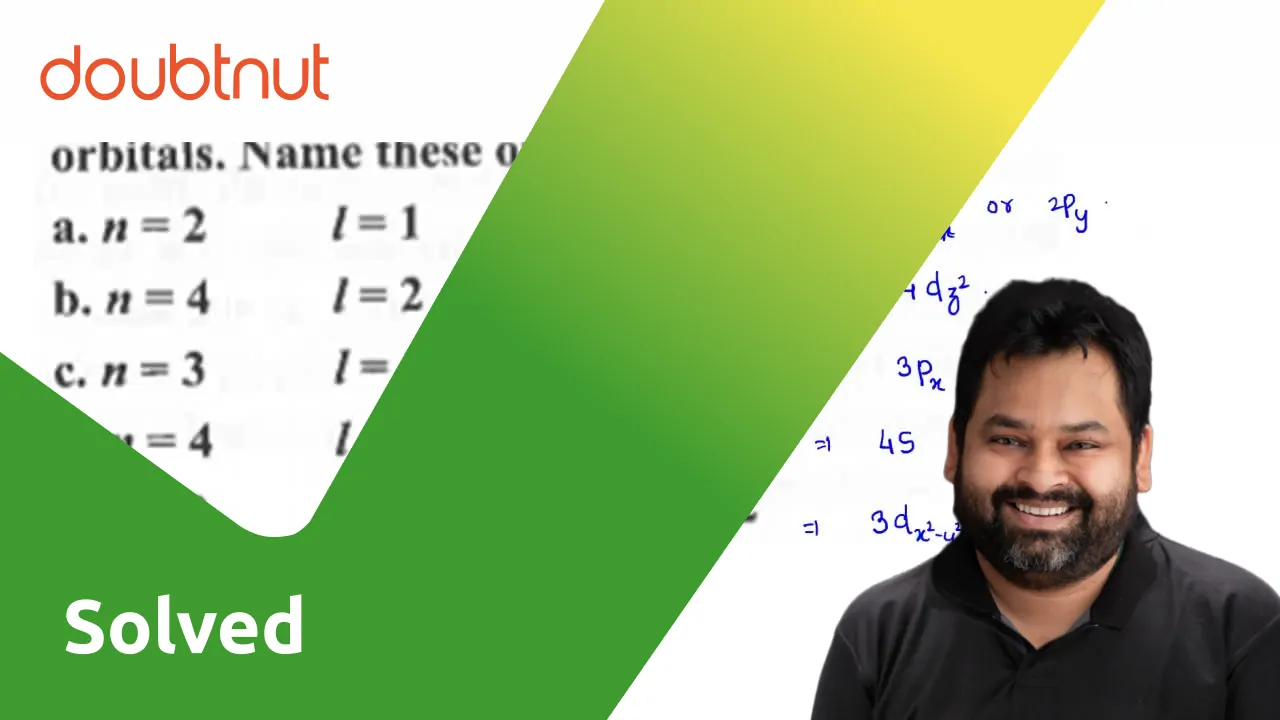Doubtnut App - Get 50 Lakh+ video solutions better than GoogleInstall Now

# Given below are the sets of quantum numbers for given orbitals .Name these orbitals a.n = 2 l = 1 m = -1<br>b.n = 4 l = 2 m = 0<br>c.n = 3 l = 1 m = +- 1<br>d.n = 4 l = 0 m = 0<br>e.n = 3 l = 2 m = +- 2

Text Solution
Verified by Experts

## a.,n=2 and l=1∴2p Also m=−1∴2pxor2py b. 4d22 c. 3pxor3py d4s e.3dx2−y2or 3dxy